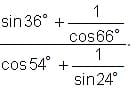CAT  >  Practice Questions Level 1: Trigonometry - 1

# Practice Questions Level 1: Trigonometry - 1 - Notes | Study Level-wise Practice Questions for CAT Preparation - CAT

 1 Crore+ students have signed up on EduRev. Have you?

This EduRev document offers 10 Multiple Choice Questions (MCQs) from the topic Trigonometry (Level - 1). These questions are of Level - 1 difficulty and will assist you in the preparation of CAT & other MBA exams. You can practice/attempt these CAT Multiple Choice Questions (MCQs) and check the explanations for a better understanding of the topic.

Question for Practice Questions Level 1: Trigonometry - 1
Try yourself:If cot (x + 27°) = tan y, then which of the following equations is true?

Question for Practice Questions Level 1: Trigonometry - 1
Try yourself:Solve for x: 2 cos (3x) - 1 = 0; where, x ∈ (0, 2π)

Question for Practice Questions Level 1: Trigonometry - 1
Try yourself:The value of cos 24° + cos 55° + cos 125° + cos 204° + cos 300° is

Question for Practice Questions Level 1: Trigonometry - 1
Try yourself:Solve the equation 2 sin2 x + 3 cos x = 0.

Question for Practice Questions Level 1: Trigonometry - 1
Try yourself:Find the value of cos2 15° - cos2 30° + cos2 45° - cos2 60° + cos2 75°.

Question for Practice Questions Level 1: Trigonometry - 1
Try yourself:Two pillars of equal height stand on either side of a road, which is 150 m wide. At a point on the road between the pillars, the angles of elevation of their tops are 60° and 30°. Find the height of the pillars.

Question for Practice Questions Level 1: Trigonometry - 1
Try yourself:If cosθ = 2cos2θ , then 2sec2θ – cos2θ + tan2θ =

Question for Practice Questions Level 1: Trigonometry - 1
Try yourself:Find the value ofQuestion for Practice Questions Level 1: Trigonometry - 1
Try yourself:The angle of elevation of a cloud from a point 60 m above a lake is 30° and the angle of depression of its image in lake is 60°. The height of the cloud is

Question for Practice Questions Level 1: Trigonometry - 1
Try yourself:Solve for the number of solution of the equation: sin(x) + cos(x) = 1 on the interval 0° ≤ x < 360°.

The document Practice Questions Level 1: Trigonometry - 1 - Notes | Study Level-wise Practice Questions for CAT Preparation - CAT is a part of the CAT Course Level-wise Practice Questions for CAT Preparation.
All you need of CAT at this link: CAT

## Level-wise Practice Questions for CAT Preparation

277 docs
 Use Code STAYHOME200 and get INR 200 additional OFF

## Level-wise Practice Questions for CAT Preparation

277 docs

### How to Prepare for CAT

Read our guide to prepare for CAT which is created by Toppers & the best Teachers

Track your progress, build streaks, highlight & save important lessons and more!

,

,

,

,

,

,

,

,

,

,

,

,

,

,

,

,

,

,

,

,

,

;